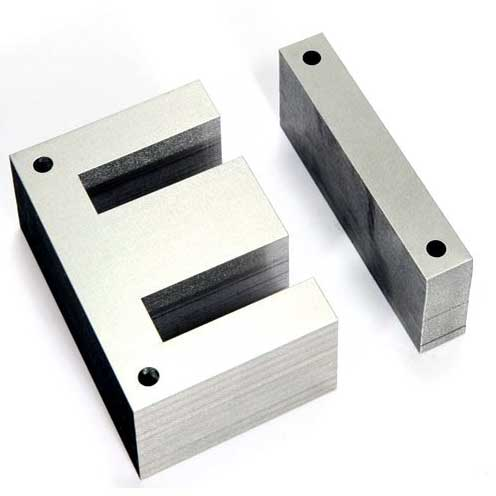MIKE'S SIMPLIFIED CHOKE THEORY

The theory is similar to the transformer theory, but a choke only has 1 winding. The turns per volt (TPV) can be calculated in the same way, but the choke has a heavy DC current flowing through it so the core WILL saturate unless the flux density is reduced massively. This is done with a gap.After the bobbin is wound with wire, the E laminations are inserted all on one side as sort of shown to the right. The I laminations are clamped together and stuck on the end of the E laminations with a gap created by a few sheets of paper. What follows is a discussion of the gap.

THE GAP

Without looking at all the hysteresis curves and permeability curves, suffice it to say that DC current through the core as in a choke or SET output transformer leads quickly to saturation of the core. So an air gap is placed to increase the reluctance. Air has a permeability of 1. The grueling calculations are below.

Reluctance (R) is the magnetic equivalent of electrical resistance. From equation (19) on the Brain Dead Transformer Theory page we can express the reluctance of the core and the reluctance of the gap and they are additive:
(1)   Rc = ℓMP/(μRμOAc)
(2)   Rg = g/μOAg            where g = width of gap, μR=1 for air
Remembering the ohm's law analogy, total R = Rc + Rg

From (18), and remembering that MMF = NI
NI = Φ(Rg + Rc)
= Φ[g/(μOAg) + ℓMP/(μRμOAc) ]
= Φ/A *[g/μO + ℓMP/(μRμO)      this assumes Ac=Ag, which is not exactly correct because of fringing.
= B*[g/μO + ℓMP/(μRμO)      from equation (3)

Divide both sides by ℓMP and remember that H = NI/ℓMP you get:
H= B * [g/(μOMP) + 1/(μRμO)]

From equation (2) H = B * 1/μ, so......
H = B * 1/μEF, where
1/μEF = 1/[g/(μOMP)+ 1/(μRμO)]

Things get a little easier for chokes (or transformers) with a gap. A typical μRμO for the core may be in the neighborhood of 10,000 while the μ for the gap is 1. For, say, EI66 laminations, a typical gap is .15mm and a typical ℓMP is maybe 12cm and A=6.8.
So, from equations (22) and (23),
Rc = 12/(10000*6.8) = 1.77x10-4
Rg = .15/6.8 = 2.2x10-3
So the reluctance Rg of the gap is 12 times the reluctance of the core, so is the overwhelming determinant of total reluctance.

EXAMPLE

Assume EI66 lamination with 31mm stack thickness. From chart a=22, so A = 3.1*2.2=6.8cm2. The magnetic path length ℓMP, as calculated way above is 12.25cm. The core has μ = 10,000 and the gap g = .15mm.
From equation (17):
Rc = ℓMP/μA = 12.25/(10000*6.8) = .0001801
Rg = g/A = .15/6.8 = .002206

So Rg is 12X as large as Rc, meaning that the total reluctance is almost entirely determined by the gap, so the quality of the core is much less important in a choke with a gap.